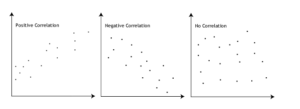# HOW TO RUN PEARSON CORRELATION TEST IN SPSS

## What is the Pearson Correlation Test?

### Using the Pearson Correlation Statistic in Research

This easy tutorial will show you how to run the Pearson Correlation test in SPSS, and how to interpret the result.

Correlation is a statistical method used to assess a possible linear association between two continuous variables. In addition, It is simple both to calculate and to interpret. (Source)

Above all, Correlation describes the strength and direction of a linear relationship between two variables.  On the other hand, the Pearson correlation coefficient is appropriate for continuous variables. Finally, we can use it when we have one continuous variable and one dichotomous variable.

First of all, Pearson correlation coefficients (r) can only take values from -1 to +1. That is to say, the sign indicates whether the correlation is positive (both variables together and declining and increasing) or negative (one variable decreasing as the other increases and vice versa).

We can categorize the type of correlation by considering as one variable increases what happens to the other variable:

• Positive correlation – the other variable has a tendency to also increase;
• Negative correlation – the other variable has a tendency to decrease;
• No correlation – the other variable does not tend to either increase or decrease.

The starting point of any such analysis should be the construction and subsequent examination of a scatterplot. For examples of negative, no, and positive correlation are as follows.### Are there guidelines for interpreting Pearson’s correlation coefficient?

Yes, We proposed the following guidelines: A Pearson correlation coefficient between 0.51 and 0.99 indicates a high correlation between variables (values above 0.80 indicate an extremely high correlation. )

### Assumptions of the Pearson Correlation Test

The assumptions for the Pearson correlation coefficient are as follows:

• Level of measurement: each variable should be continuous
• Related pairs: each participant or observation should have a pair of values
• Absence of outliers: not having outliers in either variable.
• Normality of variables: Variables should be approximately normally distributed.
• Linearity and Homoscedasticity: There is a linear relationship between two variables.

### An Example; Pearson Correlation Test

We want to examine the relationship between math test score and level of anxiety, math test score and level of stress, and level of anxiety and level of stress.

This easy tutorial will show you how to run the Pearson correlation test in SPSS, and how to interpret the result.

## GET HELP FROM US

There is a lot of statistical software out there, but SPSS is one of the most popular. If you’re a student who needs help with SPSS, there are a few different resources you can turn to. The first is SPSS Video Tutorials. We prepared a page for SPSS Tutor for Beginners. All contents can guide you through Step-by-step SPSS data analysis tutorials and you can see How to Run in Statistical Analysis in SPSS.

The second option is that you can get help from us, we give SPSS help for students with their assignments, dissertation, or research. Doing it yourself is always cheaper, but it can also be a lot more time-consuming. If you’re not the best at SPSS, then this might not be a good idea. It can take days just to figure out how to do some of the easier things in SPSS. So paying someone to do your SPSS will save you a ton of time and make your life a lot easier.

The procedure of the SPSS help service at OnlineSPSS.com is fairly simple. There are three easy-to-follow steps.

1. Click and Get a FREE Quote
2. Make the Payment
3. Get the Solution

Our purpose is to provide quick, reliable, and understandable information about SPSS data analysis to our clients.

## How to report a Pearson correlation Test results: Explanation Step by Step

### How to Report Correlation Coefficient Table in SPSS Output?

The output shows the results of the Pearson correlation coefficient.

## How to Interpret a Pearson Correlation Results in APA Style?

A Pearson correlation coefficient was computed to determine the relationship between Math test score and level of anxiety, between Math test score and level of stress, and between the level of stress and level of anxiety.

Firstly, the results indicate a non-significant positive relationship between Math test score and level of anxiety, [r(37) = .233, p = .165].  In addition, there was also non-significant negative relationship between Math test score and level of stress, [r(37) = -.279, p = .094].

In contrast, there was a significant positive relationship between level of anxiety and level of stress, [r(37) = .482, p = .000].

To sum up, as the level of anxiety increases, the level of stress increases. Therefore, we fail to reject the null hypothesis that the correlation between Math test score and level of anxiety, and between Math test score and level of stress is zero. On the other hand. we reject the null hypothesis that the correlation between the level of anxiety and the level of stress is zero.

## How Online SPSS Help Service Works

### Submit SPSS Project

Start by clicking on the GET INSTANT QUOTE button, enter the required details, and upload supporting files to submit your assignment through our user-friendly order form.

### Make The Payment

Our experts will review and update the quote for your assignment. Make the payment to start the processing, we have PayPal integration which is quick and secure.

### Get Solution

Just Relax! You will receive a high-quality result that is 100% plagiarism free within the promised deadline. We will send the solution to your e-mail as per the agreed deadline.

Hello,